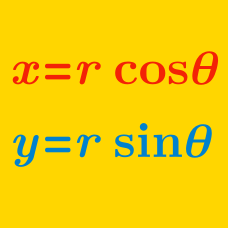Algebra

# Polar Coordinates - Multiplication

Let $z = 3(\cos(15 ^\circ) + i\sin(15 ^\circ)),$ $w = 5(\cos(54 ^\circ)+i\sin(54 ^\circ)).$ Then $$zw$$ can be expressed as $$r(\cos \alpha ^ \circ + i\sin\beta ^ \circ )$$, where $$r$$ is a real number, $$0 \leq \alpha \leq 90$$ and $$0 \leq \beta \leq 90$$. What is $$r+\alpha+\beta$$?

Let $$z_1$$ and $$z_2$$ be complex numbers such that $z_1 = 15 \left( \cos \frac{5}{12}\pi + i \sin \frac{5}{12}\pi \right),$ $z_2 = 2 \left( \cos \frac{1}{12}\pi + i \sin \frac{1}{12}\pi \right).$ The product $$z_1 z_2$$ can be expressed as $$a + bi,$$ where $$a$$ and $$b$$ are real numbers. What is the value of $$a+b?$$

Details and assumptions

$$i$$ is the imaginary number satisfying $$i^2 = -1$$.

Consider the complex numbers \begin{align} z_1 &= 5+5\sqrt{3}i, \\ z_2 &= 3\left(\cos \frac{\pi}{6}+i\sin \frac{\pi}{6}\right). \end{align} If the product $$z_1z_2$$ can be expressed as $$a+bi$$, where $$a$$ and $$b$$ are real numbers, what is $$a+b$$?

Let $$z_1, z_2$$ and $$z_3$$ be complex numbers such that \begin{align} z_1 &= 2 \left( \cos \frac{1}{12}\pi + i \sin \frac{1}{12}\pi \right), \\ z_2 &= 7 \sqrt{3} \left( \cos \frac{1}{12}\pi + i \sin \frac{1}{12}\pi \right), \\ z_3 &= 9 \left( \cos \frac{1}{6}\pi + i \sin \frac{1}{6}\pi \right). \end{align} If the product $$z_1 z_2 z_3$$ can be expressed as $$a + bi,$$ where $$a$$ and $$b$$ are real numbers, what is $$\frac{b^2}{a^2}$$?

Let $$z_1$$ and $$z_2$$ be complex numbers such that $z_1 = 10 \left( \cos \frac{1}{24}\pi + i \sin \frac{1}{24}\pi \right),$ $z_2 = 5 \sqrt{2} \left( \cos \frac{5}{24}\pi + i \sin \frac{5}{24}\pi \right).$ The product $$z_1 z_2$$ can be expressed as $$a + bi,$$ where $$a$$ and $$b$$ are real numbers. What is the value of $$a+b$$?

Details and assumptions

$$i$$ is the imaginary number that satisfies $$i^2 = -1$$.

×